## Example Questions

### Example Question #1 : How To Divide Negative Numbers

What is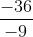?

Possible Answers: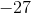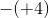45Correct answer:Explanation:

A negative number divided by a negative number always results in a positive number.divided byequals. Since the answer is positive, the answer cannot beor any other negative number.

### Example Question #2 : Negative Numbers

Solve for: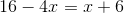Possible Answers: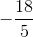Correct answer:Explanation:

Begin by isolating your variable.

Subtractfrom both sides: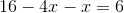, or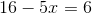Next, subtractfrom both sides: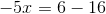, or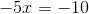Then, divide both sides by: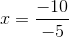Recall that division of a negative by a negative gives you a positive, therefore: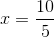or### Example Question #2 : How To Add / Subtract / Multiply / Divide Negative Numbers

Solve the following equation: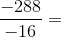Possible Answers: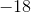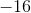Correct answer:Explanation:

The rule for dividing negative numbers is the same as for multiplying negative numbers.

If both numbers are negative, you will get a positive answer.

If either number is positive, and the other is negative, you will get a negative answer.

Therefore: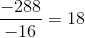### Example Question #1 : How To Add / Subtract / Multiply / Divide Negative Numbers

Choose the answer which best solves the following equation: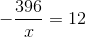Possible Answers: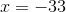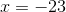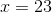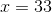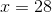Correct answer:Explanation:

To solve, first put the equation in terms of:First multiply the x to both sides.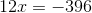Now divide by 12 to solve for x.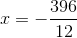Here, because one of the numbers in the equation is positive, and the other is negative, the answer must be a negative number:### All ACT Math Resources